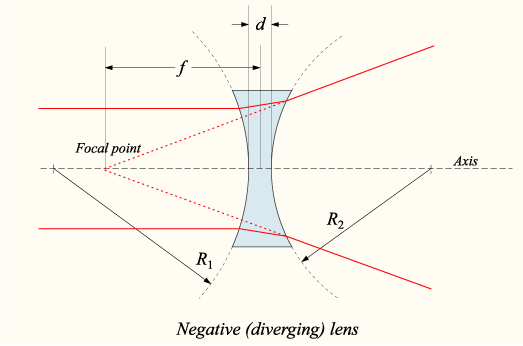# Difference Between Concave and Convex Lens

## Main Difference – Concave vs. Convex Lens

Lenses are transparent objects with a curved surface. Due to the law of refraction, light rays bend as they enter and leave the lens. By curving the lens in a specific way, it is possible to bend beams of light according to specific requirements. We draw ray diagrams to show how rays of light passing through a lens would bend. Once we draw a ray diagram, we can determine how the lens would form an image. We use several terms to describe properties of an image:

1. Inverted if the image produced is upside-down, upright if the image is “right way up”.
2. Real if the image can be projected onto a screen, and virtual  if it cannot (our eye can still see virtual images because the lens of the eye forms a real image on the retina).
3. Diminished if the image is smaller than the object, magnified if the image is larger than the object.

The main difference between concave and convex lens is that a concave lens is thinner at the centre than it is at the edges, whereas a convex lens is thicker at the centre than it is at the edges.

## What is a Concave Lens

A concave lens is a lens that is thinner at the centre than it is at the edges, i.e. it “caves” inward. A ray diagram for parallel rays of light incident on a concave lens is shown below:A ray diagram of a concave lens

Here, the rays enter the lens from the left. As they go through the lens, they diverge. Due to this, concave lenses are also called diverging lenses. Their focal points are virtual. An image formed by a concave lens is always virtual, diminished and upright:Wherever an object is placed in front of a concave lens, it forms a virtual, diminished and upright image.

## What is a Convex Lens

A convex lens is a lens that is thicker at the centre than it is at the edges, i.e. it bulges outward. A ray diagram for parallel rays incident on a concave lens is shown below:

Rays going through a convex lens converge. Therefore, convex lenses are also called converging lenses. If an object is placed in front of a convex lens, the nature of the image formed depends on where the object is placed.

If an object is placed in front of the lens at a distance less than its focal length, the image formed is upright, enlarged and virtual. This is how images are formed by magnifying glasses:

If an object is placed at a distance greater than the focal length, a real, virtual and an inverted image is produced.An object placed far away from a concave lens produces a diminished, inverted and real image.

Lenses can be constructed in many different ways. The curvature of the surface is usually achieved by grinding the surface into a smooth curved shape. The lenses in the diagrams above are curved equally on both sides. Depending on the requirement, lenses can be made into other shapes as well. The diagram below shows some of these shapes, along with their names:

We can use convex and concave lenses with interesting combinations to produce optical instruments, such as telescopes and microscopes.

## Difference Between Concave and Convex Lens

### Shape

A Concave lens is thinner in the centre.

A Concave lens is thicker in the centre.

### Effect on Parallel Rays

Concave lens diverges the parallel rays of light passing through them.

Convex lens converges the parallel rays of light passing through them.

### Image Formation

Concave lenses always produce virtual, diminished and upright images no matter where the object is kept.

The nature of the image formed by convex lenses depends on where the object is placed.

Image Courtesy

“A negative lens” by DrBob at English Wikipedia (Transferred from en.wikipedia to Commons.) [CC BY-SA 3.0], via Wikimedia Commons

“Principle of the diverging lens” by w:en:DrBob (w:en:File:Lens4.svg) [CC BY-SA 3.0], via Wikimedia Commons

“A positive lens” by DrBob at en.wikipedia (SVG version of image:lens1.png by DrBob) [CC BY-SA 3.0], via Wikimedia Commons

“image:lens3b.png” by DrBob (Own work) [CC BY-SA 3.0], via Wikimedia Commons

“Princliple of the imagining provided by the convex lens” by w:en:DrBob (w:en:File:Lens3.svg) [GFDLv1.2], via Wikimedia Commons

“Type of lenses (text labels in English)” by ElfQrin (Own work) [CC BY-SA 3.0], via Wikimedia Commons